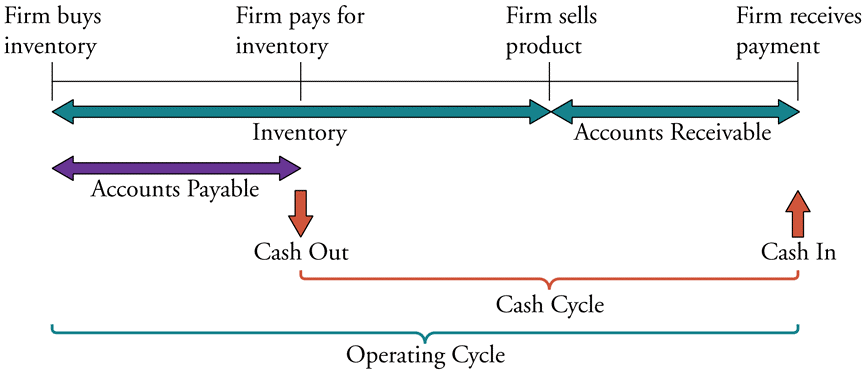# Fight Finance

#### CoursesTagsRandomAllRecentScores

Which of the following statements is NOT correct?

High risk firms in danger of bankruptcy tend to have:

Question 472  quick ratio, accounting ratio

A firm has current assets totaling $1.5b of which cash is$0.25b and inventories is $0.5b. Current liabilities total$2b of which accounts payable is $1b. What is the firm's quick ratio, also known as the acid test ratio? Which of the following is NOT a synonym of 'required return'? High risk firms in danger of bankruptcy tend to have: A firm has a debt-to-equity ratio of 25%. What is its debt-to-assets ratio? A firm has a debt-to-equity ratio of 60%. What is its debt-to-assets ratio? A firm has a debt-to-assets ratio of 20%. What is its debt-to-equity ratio? In the home loan market, the acronym LVR stands for Loan to Valuation Ratio. If you bought a house worth one million dollars, partly funded by an$800,000 home loan, then your LVR was 80%. The LVR is equivalent to which of the following ratios?

Safe firms with low chances of bankruptcy will tend to have:

The below diagram shows a firm’s cash cycle.Which of the following statements about companies’ cash cycle is NOT correct?

The Du Pont formula is:

$$\dfrac{\text{Net Profit}}{\text{Sales}} \times \dfrac{\text{Sales}}{\text{Total Assets}} \times \dfrac{\text{Total Assets}}{\text{Owners' Equity}}$$

Which of the following statements about the Du Pont formula is NOT correct?